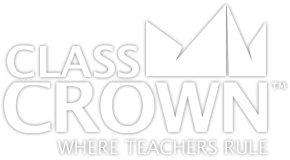# Pre-algebra Worksheets

Pre-algebra Worksheets: Pack 1
Skills included in this pack:
• Subtracting integers
• Multiplying integers
• Dividing integers
• Adding, subtracting, multiplying, and dividing integers
• Simplifying using order of operations
• Writing variable expressions
• Evaluating variable expressions
• Writing equations to represent word problems
• Completing function tables
• Solving 1-step equations by adding/subtracting
• Solving 1-step equations by multiplying/dividing
• Solving 2-step equations (includes integers)
• Writing linear functions
• Graphing linear functions
• Evaluating functions
• Writing multiplication expressions using exponents
• Evaluating exponents
• Multiplying powers
• Dividing powers
• Using scientific notation
+ See More
Free Sample Pages AvailableSelf Checking

Our math worksheets introduce a puzzle aspect to math, giving students immediate feedback as to whether or not they are solving problems correctly. If the answer to the riddle isn't spelled correctly, the student knows which problems he's made an error on.Fun Puzzle AspectImmediate FeedbackProblem Solving Motivation

Each math worksheet contains a riddle that the student solves by completeing all the problems on the worksheet. This keeps kids motivated to complete each problem so that they can find the answer to the riddle.Common Core Aligned

All our math worksheet packs are designed with Common Core in mind. That way you don’t have to worry about whether your math ciriculum is aligned or not when you incorpoate ClassCrown Riddle-Me-Worksheets in your lesson plans.High Quality Design

Each page of our math worksheets has been produced in high resolution at 144 dpi and designed in full, vibrant color for maximum quality. They look stunning whether you are printing in color or black and white.High Resolution
(144 dpi)Stunning Color & Clarity